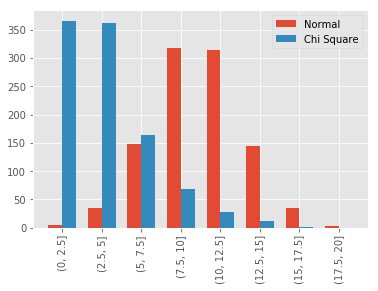## Bucketing Continuous Variables in pandas

• Post author:
• Post category:Python

# Bucketing Continuous Variables in pandas

In this post we look at bucketing (also known as binning) continuous data into discrete chunks to be used as ordinal categorical variables.

We’ll start by mocking up some fake data to use in our analysis.

We use random data from a normal distribution and a chi-square distribution.

In :
import pandas as pd
import numpy as np

np.random.seed(10)
df = pd.DataFrame({
'normal': np.random.normal(10, 3, 1000),
'chi': np.random.chisquare(4, 1000)
})


We can use the pandas function pd.cut() to cut our data into 8 discrete buckets.

The result is a series with 8 categories.

In :
pd.cut(df['normal'], 8).head()

Out:
0    (13.626, 15.833]
1     (11.42, 13.626]
2      (4.8, 7.00665]
3      (9.213, 11.42]
4     (11.42, 13.626]
Name: normal, dtype: category
Categories (8, object): [(0.369, 2.593] < (2.593, 4.8] < (4.8, 7.00665] < (7.00665, 9.213] < (9.213, 11.42] < (11.42, 13.626] < (13.626, 15.833] < (15.833, 18.0397]]

We’ll now do the same for our second distribution.

In :
pd.cut(df['chi'], 8).head()

Out:
0    (8.645, 10.784]
1     (2.229, 4.368]
2     (6.507, 8.645]
3     (4.368, 6.507]
4     (2.229, 4.368]
Name: chi, dtype: category
Categories (8, object): [(0.0738, 2.229] < (2.229, 4.368] < (4.368, 6.507] < (6.507, 8.645] < (8.645, 10.784] < (10.784, 12.922] < (12.922, 15.0607] < (15.0607, 17.199]]

Notice, however, that the buckets for the first distribution and the second distribution do not have the same start values or end values, and have different step sizes.

If we want, we can provide our own buckets by passing an array in as the second argument to the pd.cut() function, with the array consisting of bucket cut-offs.

Let’s create an array of 8 buckets to use on both distributions:

In :
custom_bucket_array = np.linspace(0, 20, 9)
custom_bucket_array

Out:
array([  0. ,   2.5,   5. ,   7.5,  10. ,  12.5,  15. ,  17.5,  20. ])

Now when we cut our data, we get buckets that are all the same range (2.5).

In :
df['normal'] = pd.cut(df['normal'], custom_bucket_array)
df['chi'] = pd.cut(df['chi'], custom_bucket_array)

Out:
chi normal
0 (7.5, 10] (12.5, 15]
1 (2.5, 5] (10, 12.5]
2 (5, 7.5] (5, 7.5]
3 (5, 7.5] (7.5, 10]
4 (2.5, 5] (10, 12.5]

We can then plot this data to show the distribution densities using the same buckets for both distributions.

In :
import matplotlib.pyplot as plt
%matplotlib inline

plt.style.use('ggplot')

a = df.groupby('normal').size()
b = df.groupby('chi').size()

categories = df['normal'].cat.categories
ind = np.array([x for x, _ in enumerate(categories)])
width = 0.35
plt.bar(ind, a, width, label='Normal')
plt.bar(ind + width, b, width,
label='Chi Square')

plt.xticks(ind + width / 2, categories)
plt.legend(loc='best')
plt.xticks(rotation = 90)
plt.show()